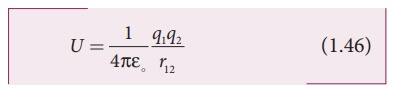Home | | Physics 12th Std | Electrostatic potential energy for collection of point charges

# Electrostatic potential energy for collection of point charges

Physics : Electrostatics: Electrostatic Potential and Potential Energy : Electrostatic potential energy for collection of point charges

Electrostatic potential energy for collection of point charges

The electric potential at a point at a distance r from point charge q1 is given byThis potential V is the work done to bring a unit positive charge from infinity to the point. Now if the charge q2 is brought from infinity to that point at a distance r from q1, the work done is the product of q2 and the electric potential at that point. Thus we have

W = q2V

This work done is stored as the electrostatic potential energy U of a system of charges q1 and q2 separated by a distance r. Thus we haveThe electrostatic potential energy depends only on the distance between the two point charges. In fact, the expression (1.45) is derived by assuming that q1 is fixed and q2 is brought from infinity. The equation (1.45) holds true when q2 is fixed and q1 is brought from infinity or both q1 and q2 are simultaneously brought from infinity to a distance r between them.

Three charges are arranged in the following configuration as shown in Figure 1.30.To calculate the total electrostatic potential energy, we use the following procedure. We bring all the charges one by one and arrange them according to the configuration as shown in Figure 1.30.

(i) Bringing a charge q1 from infinity to the point A requires no work, because there are no other charges already present in the vicinity of charge q1.

(ii) To bring the second charge q2 to the point B, work must be done against the electric field created by the charge q1. So the work done on the charge q2 is W = q2 V 1B. Here V1B is the electrostatic potential due to the charge q1 at point B.Note that the expression is same when q2 is brought first and then q1 later.

(iii) Similarly to bring the charge q3 to the point C, work has to be done against the total electric field due to both charges q1 and q2. So the work done to bring the charge q3 is = q3 (V1C + V2C). Here V1C is the electrostatic potential due to charge q1 at point C and V2C is the electrostatic potential due to charge q2 at point C.

The electrostatic potential is(iv) Adding equations (1.46) and (1.47), the total electrostatic potential energy for the system of three charges q1, q2 and q3 isNote that this stored potential energy U is equal to the total external work done to assemble the three charges at the given locations. The expression (1.48) is same if the charges are brought to their positions in any other order. Since the Coulomb force is a conservative force, the electrostatic potential energy is independent of the manner in which the configuration of charges is arrived at.

EXAMPLE 1.15

Four charges are arranged at the corners of the square PQRS of side a as shown in the figure.(a) Find the work required to assemble these charges in the given configuration. (b) Suppose a charge q′ is brought to the center of the square, by keeping the four charges fixed at the corners, how much extra work is required for this?Solution

(a) The work done to arrange the charges in the corners of the square is independent of the way they are arranged. We can follow any order.

(i) First, the charge +q is brought to the corner P. This requires no work since no charge is already present, WP = 0

(ii) Work required to bring the charge –q to the corner Q = (-q) x potential at a point Q due to +q located at a point P.

WQ = −q × 1/4πε . q/ = − 1/4πε  .  q2/a(iii) Work required to bring the charge +q to the corner R= q × potential at the point R due to charges at the point P and Q.(iv) Work required to bring the fourth charge –q at the position S = q × potential at the point S due the all the three charges at the point P, Q and R(b) Work required to bring the charge q′ to the center of the square = q′ × potential at the center point O due to all the four charges in the four corners

The potential created by the two +q charges are canceled by the potential created by the -q charges which are located in the opposite corners. Therefore the net electric potential at the center O due to all the charges in the corners is zero.

Hence no work is required to bring any charge to the point O. Physically this implies that if any charge q′ when brought close to O, then it moves to the point O without any external force.

Study Material, Lecturing Notes, Assignment, Reference, Wiki description explanation, brief detail
12th Physics : Electrostatics : Electrostatic potential energy for collection of point charges |